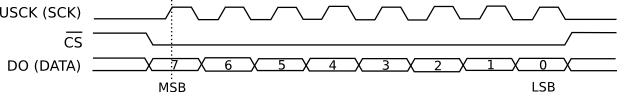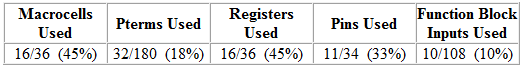Starting Electronics needs your help! Please make a donation to help cover our hosting and other costs. Click the donate button to send a donation of any amount.# Tutorial 15: VHDL SPI Receiver / Output Expander

Created on: 6 March 2013

A SPI receiver is written in VHDL and implemented on a CPLD as a SPI slave device. This allows the CPLD to act as an output expander, turning two SPI lines and a chip select line into eight output lines (8 digital outputs).

SPI (Serial Peripheral Interface) is a four-wire synchronous serial bus. In this example, only three wires are used (data, clock and chip select) as data is only being received by the VHDL SPI receiver from a microcontroller (the micro is the SPI master).

This is the first example of microcontroller to CPLD interfacing on this VHDL course so far. The microcontroller was previously only used to generate a clock pulse for the CPLD.

This video shows the hardware, microcontroller software and VHDL code for this tutorial in action:

Can't see the video? View on YouTube →

## Hardware

All the hardware is in place if using the home built Xilinx CPLD board.

### Microcontroller

The ATtiny2313 microcontroller on the home built Xilinx CPLD board is used to send data over the SPI lines. The ATtiny2313 has a USI (Universal Serial Interface) that is used to implement the SPI port. The USI does not have a chip select line (usually called slave select (SS) on SPI), so this is implemented by using a spare microcontroller pin.

If you are using a different CPLD or FPGA board, then hook up an ATtiny2313 to it using a breadboard. If you want to use a different microcontroller or a separate microcontroller board, then you will need to write the SPI software for it yourself.

### Interface

The circuit diagram for the home built Xilinx CPLD board shows the connections between the ATtiny2313 microcontroller and the CPLD.

The diagram below shows just the SPI connections for transmitting from the microcontroller to CPLD:AVR (ATtiny2313) to CPLD (XC9536XL) SPI Transmit Connections

All the lines shown in the diagram are outputs from the microcontroller.

## Timing Diagram

The timing diagram for the SPI signals (transmit only) generated by the ATtiny2313 AVR microcontroller are shown below.SPI Timing Diagram – Transmit Only

The SPI clock phase and polarity can usually be configured in software for the microcontroller that has a SPI peripheral. The timing diagram above shows how SPI is configured in software in the ATtiny2313 microcontroller for this tutorial.

All serial data is valid on the rising edge of the clock (USCK).

## Microcontroller SPI Software

To generate the above SPI outputs from the ATtiny2313 microcontroller, you will need to compile and load the following C program, or download the Atmel Studio 6 project files which includes the source and hex files.

```#include <avr/io.h>

void SPI_tx(unsigned char);
void Delay(void);

int main(void)
{
// enable the DO and USCK pins as outputs in the DDRB register
DDRB |= ((1 << PB6) | (1 << PB7));
DDRB |= (1 << PB4);       // PB4 as chip select line /CS

PORTB |=  (1 << PB4); // make /CS line inactive (high)

while (1) {
SPI_tx(0x55);
Delay();
SPI_tx(0xF0);
Delay();
SPI_tx(0xC0);
Delay();
SPI_tx(0xC3);
Delay();
}
}

void SPI_tx(unsigned char data_byte)
{
USIDR = data_byte;              // data to send
// clear the USI counter overflow flag and USI counter value
USISR = (1 << USIOIF);
PORTB &=  ~(1 << PB4);    // enable /CS (low)
while (!(USISR & (1 << USIOIF))) { // wait for counter to overflow
// set up the USI in 3 wire mode, pos. edge shift, S/W clock strobe
// toggle clock pin
USICR = ((1 << USIWM0) | (1 << USICS1) | (1 << USICLK) | (1 << USITC));
}
PORTB |=  (1 << PB4); // disable /CS (high)
}

void Delay(void)
{
volatile unsigned int del = 40000;
while(del--);
}
```

This program must be loaded to the ATtiny2313 microcontroller. The program sends out four bytes continuously (0x55, 0xF0, 0xC0 and 0xC3), each with a different value, over the SPI port.

The idea is for the SPI receiver implemented on the CPLD to receive each byte from the SPI lines and display it on eight LEDs.

## Thinking Through the VHDL Design

### Clock Pulse and Data

In this VHDL CPLD course, we have already seen how to run VHDL code when a clock edge occurs by using a VHDL process. It should not be too difficult to sample the data line on every clock pulse rising edge.

Eight clock pulses are sent from the SPI master (the microcontroller) for every byte of data sent. Valid data can be found on the SPI data line (DO – data out) from the microcontroller on every rising clock edge.

To store the received byte, each bit can be shifted into a register on each rising edge of the clock pulse – we have already seen how to shift in VHDL.

### Synchronization

It would be difficult to make sure that the microcontroller and CPLD stay in sync if just a clock and data line were used. Any pulse on the clock line during power up could immediately put the microcontroller and CPLD out of sync – the CPLD would not know if it was the first data bit of a byte or any other bit in the byte when the microcontroller starts sending data.

The chip select (CS) (usually called slave select (SS) in SPI devices) keeps the microcontroller and CPLD synchronized. On the falling edge of CS, we know that a new byte will be coming from the microcontroller. On the rising edge of CS, we know that we have received a byte from the microcontroller.

### Data Buffering

If the register used for shifting in the serial data were connected directly to the LEDs, the LEDs would all appear to be on unless a byte with a value of zero were sent. This is because we would be seeing the data being shifted at full speed across all the LEDs.

The received data byte needs to be buffered in order to prevent shifting data being displayed on the LEDs. The LEDs can then be connected to the buffer register. The buffer register can then be connected to the LEDs and updated only on the rising edge of CS when the new byte of data has been fully received.

Two VHDL code examples (SPI_rx2 and SPI_rx3) are shown below and each explained in turn:

### SPI Receiver VHDL Code – Example 1

The first VHDL code example – SPI_rx2_top.vhd:

```library IEEE;
use IEEE.STD_LOGIC_1164.ALL;

entity SPI_rx2_top is
Port ( SCK  : in  STD_LOGIC;    -- SPI input clock
DATA : in  STD_LOGIC;    -- SPI serial data input
CS   : in  STD_LOGIC;    -- chip select input (active low)
LED  : inout STD_LOGIC_VECTOR (7 downto 0));
end SPI_rx2_top;

architecture Behavioral of SPI_rx2_top is
signal dat_reg : STD_LOGIC_VECTOR (7 downto 0);
begin

process (SCK)
begin
if (SCK'event and SCK = '1') then  -- rising edge of SCK
if (CS = '0') then             -- SPI CS must be selected
-- shift serial data into dat_reg on each rising edge
-- of SCK, MSB first
dat_reg <= dat_reg(6 downto 0) & DATA;
end if;
end if;
end process;

-- only update LEDs when not shifting (CS inactive)
LED <= dat_reg when (CS = '1') else LED;

end Behavioral;
```

#### Getting the Data Bits

The VHDL process in the above code gets a data bit on every rising edge of the SPI clock. A data bit will only be read if the CS line is active (low) to prevent any spurious signals on the clock line causing the microcontroller and CPLD to get out of sync.

#### Shifting the Data into a Register

The data bits that occur at each rising edge of the clock pulse are shifted into the dat_reg register using the following line of VHDL code:

```dat_reg <= dat_reg(6 downto 0) & DATA;
```

The shifting operation is done by moving the bottom 7 bits (6 down to 0) left by one bit in the dat_reg register. At the same time the valid data on the DATA line is appended to the seven lower bits that are being shifted. The bit from the DATA line is appended by using the VHDL concatenation operator (&).

This effectively shifts the bottom seven bits of dat_reg left by one and puts the new data bit from the DATA line into bit 0 of dat_reg.

#### Displaying the Data Bytes

The received data bytes are displayed on the LEDs by updating a received byte only when the CS line is inactive (high) by using the VHDL when – else construct:

```LED <= dat_reg when (CS = '1') else LED;
```

In the entity part of the VHDL code, LED was made inout so that it can be read back in the above line of code (after the else).

The above line of code creates a latch which is considered bad programming in VHDL. The VHDL compiler tools will also bring up a warning that a latch has been created.

The next VHDL example shows how to get rid of the latch and use a register for storing the received bytes for displaying on the LEDs.

### SPI Receiver VHDL Code – Example 2

The second VHDL code example – SPI_rx3_top.vhd:

```library IEEE;
use IEEE.STD_LOGIC_1164.ALL;

entity SPI_rx3_top is
Port ( SCK  : in  STD_LOGIC;    -- SPI input clock
DATA : in  STD_LOGIC;    -- SPI serial data input
CS   : in  STD_LOGIC;    -- chip select input (active low)
LED  : out STD_LOGIC_VECTOR (7 downto 0) := X"FF");
end SPI_rx3_top;

architecture Behavioral of SPI_rx3_top is
signal dat_reg : STD_LOGIC_VECTOR (7 downto 0);
begin

process (SCK)
begin
if (SCK'event and SCK = '1') then  -- rising edge of SCK
if (CS = '0') then             -- SPI CS must be selected
-- shift serial data into dat_reg on each rising edge
-- of SCK, MSB first
dat_reg <= dat_reg(6 downto 0) & DATA;
end if;
end if;
end process;

process (CS)
begin
if (CS'event and CS = '1') then
-- update LEDs with new data on rising edge of CS
LED <= not dat_reg;
end if;
end process;

end Behavioral;
```

In the above code, firstly LED is made an output only in the entity part of the VHDL code:

```LED  : out STD_LOGIC_VECTOR (7 downto 0) := X"FF"
```

LED is also set to an initial value of FFh which will switch all the LEDs off on the home built Xilinx CPLD board as the wiring on the board effectively inverts the CPLD signals to the LEDs.

By using a process and triggering off the rising edge of CS, a register instead of a latch is implemented which buffers the received bytes from the SPI port. The LED register will now only be updated when valid data is available from the SPI port.

The not operator is used in the above example when moving a data byte to the LED register – again to compensate for the inverting LEDs on the home built Xilinx CPLD board.

### CPLD Resources Used

It is interesting to note that the first example of the SPI receiver that implements a latch uses more CPLD Pterms than the second example as shown in the images below.CPLD Resources Used in SPI Receiver Example 1CPLD Resources Used in SPI Receiver Example 2

### All Projects Source Code

The source code for the microcontroller and both VHDL project examples can be downloaded below:

Microcontroller source code (AVR Studio 6 project): SPI_tx_ATtiny2313.zip (15.2 kB)

VHDL example 1 (ISE project): SPI_rx2.zip (719.9 kB)

VHDL example 2 (ISE project): SPI_rx3.zip (715.7 kB)